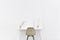# Python NamedTuples: How They Help You to Write Readable Code

With easy to follow examples for beginnersPhoto by Bench Accounting on Unsplash

Python comes with many specialised data types. In this article, I will try to explain NamedTuples by providing easy-to-follow examples for the beginners.

# What is ‘NamedTuple’ Data Type?

NamedTuple is basically an extension of the Python built-in tuple data type. Here is how doc.python.org defines the NamedTuples;

Named tuples assign meaning to each position in a tuple and allow for more readable, self-documenting code. They can be used wherever regular tuples are used, and they add the ability to access fields by name instead of position index

To understand NamedTuples further, let’s first recall what the Python tuples are.

Python tuples are;

• immutable objects: values inside a tuple object cannot be revised after creation.
• sequence type data structures that allow accessing items contained with the 0-based integer positional index.
`a_tuple = tuple()a_tuple = (1,2,3,4,5)print (a_tuple) #accessing the element with only positional indexa_tuple = 10 #tuples are immutable so you can not revise themOutput:3TypeError: 'tuple' object does not support item assignment`

The downsides of the tuples;

• You can access the elements of tuple only with the positional index. If you need to get a specific item from a tuple object, you should know the index number of that specific item. In addition, using indexing in such cases will harm the readability of your code.
• While using tuples to store data that a function returns, you have to ensure the correct order of the elements.

Let’s understand how NamedTupes solve these issues in the following chapter.

# How They Help Writing Clean Code

NamesTuples come to solve these issues, here is how;

• Each element contained in NamedTuples can be accessed through a unique and human-readable identifier. So you don’t have to remember integer indexes.
• Since you can use human-readable identifier instead of integer positional index, code readability increases and you can better express your intentions.
• While using NamedTuples to store data that a function returns, you don’t need to consider the order of the elements.

Let’s see in the code snippets below, how they solve above-mentioned issues;

`from collections import namedtupleimport mathPolar_Coordinate = namedtuple('Polar_Coordinate', 'r theta')def convert_cartesian_to_polar(x, y):  #Calculate Polar Cooordinates  r0 = (x**2 + y**2)**0.5  theta0 = math.atan2(y,x)  #Convert from radians to degrees  theta0 = math.degrees(theta0)    return Polar_Coordinate(theta=theta0, r=r0)box_position = convert_cartesian_to_polar(10, 10)print(box_position)print(box_position.r)print(box_position.theta)print(type(box_position))Output:Polar_Coordinate(r=14.142135623730951, theta=45.0) 14.142135623730951 45.0 <class '__main__.Polar_Coordinate'>`

As you can see above, with the help of the NamedTuples we can access the items with their names, which are r and theta respectively.

# Key Takeaways and Conclusion

In this short article, I have explained Python namedtuples and how they help you to write readable code. The key takeaways are;

• You can access the elements of tuple only with the positional index. If you need to get a specific item from a tuple object, you should know the index number of that specific item. NamedTuples are introduced to solve this problem.
• Each element contained in NamedTuples can be accessed through a unique and human-readable identifier. So you don’t have to remember integer indexes.
• Since you can use human-readable identifier instead of integer positional index, code readability increases and you can better express your intentions.

I hope you have found the article useful and you will start using Python namedtuples in your own code.

Machine Learning and Data Science Enthusiasts, Automotive Engineer, Mechanical Engineer, https://www.linkedin.com/in/erdem-isbilen/

## More from Erdem Isbilen

Machine Learning and Data Science Enthusiasts, Automotive Engineer, Mechanical Engineer, https://www.linkedin.com/in/erdem-isbilen/